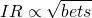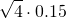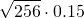# Fundamental Law of Active Management

The fundamental law of active management is the observation that the information ratio of any trading strategy is proportional to the square root of the number of independent bets made per year. The law was first articulated by Richard Kahn. On this page, we discuss the famous law of active management, provide the actual formula, and discuss a number of numerical examples.

## Law of active management definition

As we stated above, the fundamental law of active management argues that, as a strategy makes more independent bets, the performance of the strategy improves on a risk-adjusted basis. In particular, the ‘information ratio’ increases. The law states that risk-adjusted performance is proportional to the square root of the number of independent bets. Algebraically:Where IR is the information ratio, and # bets is het number of independent bets per year. This means that if an investor is trading once a week, then the number of bets equals 52. The law of active management gives us some idea of how profits can be expected to vary with trading speed. Clearly, we first need to determine the information ratio of a strategy before we can analyse the impact of increasing the trading speed. We provide an Excel that calculates the information ratio here.

## Fundamental law of active management example

Now let’s put the law into practice. Suppose we have a trading strategy that we expect will earn an information ratio (IR) of 0.15 year and that requires one trade per year. In that case, if we can make four ‘bets’ a year, then we can expect to earn an information ratio equal toor 0.3 per year. Next, suppose we can make a bet once a day. In that case the information ratio we can obtain equalsor 2.4, which is really high.

## Flaws

The above result is only theoretical. In particular, the law assumes that the ‘skill’ of the investor is constant and independent of the holding period. In other words, the law assumes that a long-term investor can increase the frequency of his or her strategy to that of a day trading strategy. Clearly, this doesn’t make sense. Second, the fundamental law of active management ignores transaction costs. As the trading frequency increases, transaction costs can have a really big impact.

## Summary

Despite these flaws, it’s important to note the most important implication. In particular, the law of active management implies that, if you apply the same strategy successfully across different markets, then the performance can increase considerably.